Difference between revisions of "Talk:Capillary waves"

Thermal capillary waves

Hello, now I'm writing the same article for Russian wikipedia. While I was deducing the expression for mean square amplitude I found my result to be two times less than common one (that is in Molecular Theory of Capillarity and refered to in many articles). Could you please tell me weather I am right or not.

I claim that the mean energy of each mode is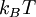$k_B T$ rather than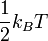$\frac{1}{2} k_B T$. That's because each mode has to degrees of freedom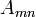$A_{mn}$ and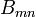$B_{mn}$, since each wave is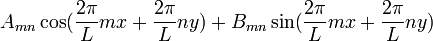$A_{mn} \cos(\frac{2\pi}{L}mx+\frac{2\pi}{L}ny) + B_{mn} \sin(\frac{2\pi}{L}mx+\frac{2\pi}{L}ny)$, with the energy of each mode proportional to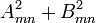$A_{mn}^2+B_{mn}^2$. This obviously lead to the mean energy of each mode to be$k_B T$. That was the real notation and now lets turn to the complex notation.

Each mode with the fixed wave vector is presented as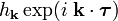$h_\mathbf{k} \exp(i \; \mathbf{k} \cdot \boldsymbol{\tau})$,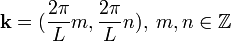$\mathbf{k}=(\frac{2 \pi}{L}m, \frac{2 \pi}{L}n), \; m,n \in \mathbb{Z}$ — wave vector,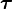$\boldsymbol{\tau}$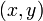$(x,y)$ vector. The energy is proportional to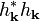$h_\mathbf{k}^*h_\mathbf{k}$ (indeed it is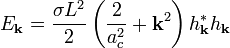$E_\mathbf{k}=\frac{\sigma L^2}{2} \left( \frac{2}{a_c^2} + \mathbf{k}^2 \right) h^*_\mathbf{k} h_\mathbf{k}$). According to equipartition: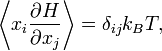$\left \langle x_i \frac{\partial H}{\partial x_j} \right \rangle = \delta_{ij} k_B T,$

we obtain: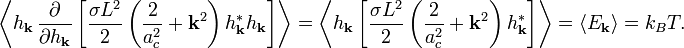$\left \langle h_\mathbf{k} \, \frac{\partial}{\partial h_\mathbf{k}} \left[ \frac{\sigma L^2}{2} \left( \frac{2}{a_c^2} + \mathbf{k}^2 \right) h^*_\mathbf{k} h_\mathbf{k} \right] \right \rangle = \left \langle h_\mathbf{k} \left[ \frac{\sigma L^2}{2} \left( \frac{2}{a_c^2} + \mathbf{k}^2 \right) h^*_\mathbf{k} \right] \right \rangle = \left \langle E_\mathbf{k} \right \rangle = k_B T.$

And again we get the same result. What do you think of it? Is there a mistake? Please help, I'm really stuck with it. Grigory Sarnitskiy. 91.76.179.101 19:45, 30 January 2009 (CET)

A quick comment

I may be wrong, but looking at your derivation it seems the boundary conditions are not correctly described. If the system is fixed to some immobile frame, only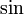$\sin$ terms should appear in the modes, not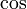$\cos$. If, on the other hand, periodic boundary conditions are applied, the opposite applies: only$\cos$, not$\sin$. This may explain the factor of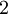$2$ that's missing... but I still have to think more carefully about this. --Dduque 09:58, 3 February 2009 (CET)